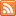# Activity 1: Learn By Doing Category“Learn By Doing” activities for our course materials.

## Learn by Doing – Normal Random Variables

Published: Feb 8th, 2013

The purpose of this interactive activity is to: Get a better feel for the behavior of normal random variables. Check the accuracy of the Standard Deviation Rule. Do some other […]

## Learn by Doing – Using the Standard Deviation Rule

Published: Feb 8th, 2013

This document is linked from Normal Random Variables.

## Learn by Doing – Shapes of Binomial Distributions

Published: Feb 7th, 2013

Using the online calculator, answer the following questions: Online Binomial Calculator:  Not JAVA Version This document is linked from Binomial Random Variables.

## Learn by Doing – Binomial Application

Published: Feb 3rd, 2013

The purpose of this activity is to gain experience making probabilistic decisions using binomial random variables. Background In vitro fertilization is becoming more and more common these days. Suppose each […]

## Learn by Doing – Binomial Probabilities

Published: Feb 3rd, 2013

The purpose of this activity is to give guided practice at solving problems involving binomial random variables and to teach how the same probabilities can be found using an online […]## Learn by Doing – Unusual or Not?

Published: Feb 3rd, 2013

Recall the probability distribution for changing majors. We have made the following calculations for the mean and standard deviation. For some extra practice, feel free to verify our calculations. This […]

## Learn by Doing – Comparing Probability Distributions #3

Published: Feb 3rd, 2013

Xavier’s Production Line: Yves’ Production Line: Hint This document is linked from Discrete Random Variables.## Learn by Doing – Comparing Probability Distributions #2

Published: Feb 2nd, 2013

Keeping in mind that the mean describes where a histogram is centered, and the standard deviation describes spread by reporting the typical distance of values from their mean, match the random […]

## Learn by Doing – Comparing Probability Distributions #1

Published: Feb 2nd, 2013

Xavier’s Production Line: Yves’ Production Line: Hint This document is linked from Discrete Random Variables.## Learn by Doing – Probability Distributions

Published: Feb 2nd, 2013

The probability distribution of the random variable X is represented by the following histogram. In the following questions, fill in the probability distribution table values labeled below consistent with the […]